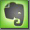Voltage Drop in Power Cables

The voltage drop in power cables is caused by the resistance of the cable material (usually copper or aluminum). It depends of the cable size (cross section) and the current flow (power consumption).

The voltage drop in 230V systems should be:
1. from the house entry point to the consumer outlet: less than 4% (German Standard VDE 0100-520)
2. from the amperage counter to the consumer outlet: less than 3% (German Standard DIN 18015-1).

The voltage drop can be calculated:voltage drop voltage drop (cos(phi)=1voltage drop in percent
l: cable length (from source to destination)  [m]
P: power consumption  [Watt]
æ: conductivity (copper at 20ºC=56)
A: cable cross section  [mm²]
U: nominal voltage  [V] (e.G. 230V)

Example 1:
l: cable length from power distribution to outlet = 50m
P: power consumption at outlet = 200W
æ: (copper) = 56
A: power cable with cross section 2,5mm²
U: nominal power 230V
cos(phi) = 1
voltage drop: ( 2 * 50 * 200 * 1 ) / ( 56 * 2,5 * 230 ) = 0,6 V
The voltage will drop in the power line from source to destination from 230V to 229,4V   (230V - 0,6V).

Example 2:
l: cable length from power distribution to outlet = 200m
P: power consumption at outlet = 1.000W
æ: (copper) = 56
A: power cable with cross section 2,5mm²
U: nominal power 230V
cos(phi) = 1
voltage drop: ( 2 * 200 * 1000 * 1 ) / ( 56 * 2,5 * 230 ) = 12,4 V
The voltage will drop in the power line from source to destination from 230V to 217,6V   (230V - 12,4V).Download: Excel File with Voltage Drop Calculations